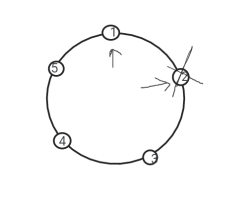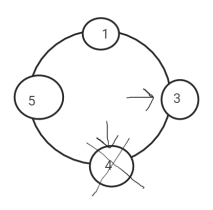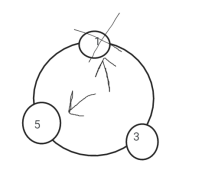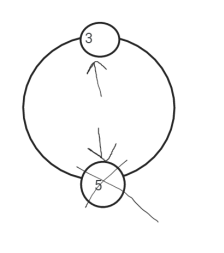# Josephus Problem

Problem Statement: There are n people standing in a circle waiting to be executed. The counting out begins at some point in the circle and proceeds around the circle in a fixed direction. In each step, a certain number of people are skipped and the next person is executed. The elimination proceeds around the circle (which is becoming smaller and smaller as the executed people are removed), until only the last person remains, who is given freedom. Given the total number of person n and a number k which indicates that k-1 persons are skipped and the kth person is killed in a circle. The task is to choose the place in the initial circle so that you are the last one remaining and so survive.

Examples:

```Example 1:
Input: n = 5 and k = 2
Output: The safe position is 3
Explanation:  Firstly, the person at position 2 is killed, then person at position 4 is killed, then person at position 1 is killed. Finally, the person at position 5 is killed. So the person at position 3 survives.

Example 2:
Input:  n = 7 and k = 3
Output: The safe position is 4
Explanation: The persons at positions 3, 6, 2, 7, 5, 1 are killed in order, and the person at position 4 survives. ```

### Solution:

Disclaimer: Don’t jump directly to the solution, try it out yourself first.

### Approach:

So, this one is a very famous theoretical problem in computer science. Let me explain one example for a better understanding.

For N = 5 , K = 2

In the first round, we will start from 1 and count 2 starting from 1, and the 2nd person will be killed.Now 4 people are left, for the next round, so we will start from 3 now. So now starting from 3, count 2, and the 4th person will be killed.

Now 3 people are left and we will again start counting from 5.

Now 3 people are left and we will again start counting from 5.

Now 3 people are left and we will again start counting from 5.Now 3 people are left and we will again start counting from 5. Counting 2 from 5, the 1st person will be killed.So now 2 people are left. We will start counting from 3. Counting 2 from 3, the 5th person will be killed.So now we are left with only one person, and that person will survive.

So how do think of the solution?

We are just killing the people and reducing the array size and waiting for only one single element. means we are repeating this same process again and again. We will solve this question using recursion.

So how to apply recursion here….

If we see in the above example, taking the numbers in an array.

### Flow of recursion

1. Base case – the base case will be when there will be only 1 element left in the array, which means that was the person who survived.
2. Small calculation – here we can notice that if we are starting from the 0th index , then we are going to the 1st index for the count of 2 . So while calculating , we need to go to index+k-1.and then erase that element and maintain the numbering of persons as it is but their positions/indexes will change.
3. Recursive Call – Then we will just make a recursive call on the rest of the array.

Code:

## C++ Code

``````#include<bits/stdc++.h>
using namespace std;

void solve(vector < int > person, int k, int index, int & ans) {
if (person.size() == 1) { //base case
ans = person; //if last element is left
return;
}
index =(index + k) % person.size(); //finding the person to be killed
person.erase(person.begin() + index); //to erase that person
solve(person, k, index, ans); //recursive call
return;
}
int main() {
int n = 7 , k = 3 ;
vector < int > person; //to store the person numbering
for (int i = 1; i <= n; i++) {
person.push_back(i);
}
int ans = -1; //to store the safest place
solve(person, k - 1, 0, ans);
cout<<"The safe position is: "<<ans<<endl;
}
``````

Output:

The safe position is: 4

Time Complexity: O(n^2), because the time complexity of the erase function is O(n) and we are using it n times

Space Complexity: O(n), vector for storing the elements.

## Java Code

``````import java.util.*;

class TUF{
static void solve(ArrayList<Integer> person, int k, int index, int ans[]) {
if (person.size() == 1) { //base case
ans = person.get(0); //if last element is left
return;
}
index = (index + k) % person.size(); //finding the person to be killed
person.remove(index); //to erase that person
solve(person, k, index, ans); //recursive call
return;
}

public static void main(String args[]) {
int n=7, k=3;
ArrayList<Integer> person=new ArrayList<>(); //to store the person numbering
for (int i = 1; i <= n; i++) {
}
int ans[]=new int;
ans=-1; //to store the safest place
solve(person, k - 1, 0, ans);
System.out.println("The safe position is: "+ans);
}
}``````

Output:

The safe position is: 4

Time Complexity: O(n^2), because the time complexity of the erase function is O(n) and we are using it n times

Space Complexity: O(n), vector for storing the elements.

Special thanks to Ishita Dhiman for contributing to this article on takeUforward. If you also wish to share your knowledge with the takeUforward fam, please check out this article